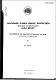## Solutions of the relativistic two-body problem II quantum mechanics1972-08
##### Publisher
Australian Atomic Energy Commission
##### Abstract
This second paper of a series discusses the formulation of the quantum mechanical equivalent of the relative time classical theory put forward in Part 1. The relativistic wave function is derived and a covariant addition theorem put forward which allows a covariant scattering theory to be established. The free particle eigenfunctions are not plane waves and a covariant partial wave analysis is given. A means is given by which wave functions which yield probability densities in 4-space can be converted to ones yielding the equivalent 3-space density. Bound states are considered and covariant analogues are given of the harmonic oscillator potential, Coulomb potential, the square well potential, and two-body fermion interactions. RESEARCH ARTICLE Previous Next Contents Vol 25 (2) Solutions of the Relativistic Two-Body Problem. II. Quantum Mechanics JL Cook Australian Journal of Physics 25(2) 141 - 166 Published: 1972 Abstract This paper discusses the formulation of a quantum mechanical equivalent of the relative time classical theory proposed in Part I. The relativistic wavefunction is derived and a covariant addition theorem is put forward which allows a covariant scattering theory to be established. The free particle eigenfunctions that are given are found not to be plane waves. A covariant partial wave analysis is also given. A means is described of converting wavefunctions that yield probability densities in 4-space to ones that yield the 3-space equivalents. Bound states are considered and covariant analogues of the Coulomb potential, harmonic oscillator potential, inverse cube law of force, square well potential, and two-body fermion interactions are discussed.
##### Keywords
Quantum mechanics, Relativity theory, Scattering, Angular momentum, Bosons, Harmonic potential
##### Citation
Cook, J. L. (1972). Solutions of the relativistic two-body problem II quantum mechanics. (AAEC-TM-602). Lucas Heights, NSW: Australian Atomic Energy Commission.